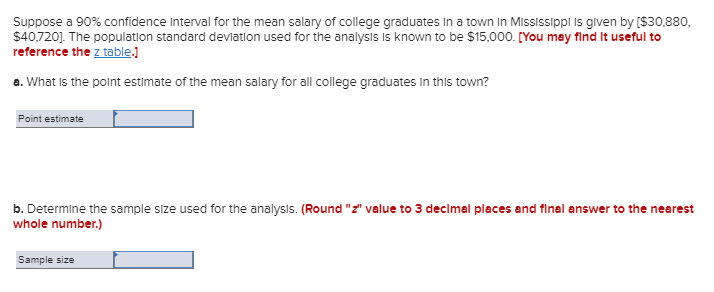(Solved): Suppose A 90% Confidence Interval For The Mean Salary Of College Graduates In A Town In Mississippi ...Suppose a 90% confidence interval for the mean salary of college graduates in a town in Mississippi is given by (\$30,880, \$40,720]. The population standard deviation used for the analysis is known to be \$15,000. [You may find it useful to reference the z table.) a. What is the point estimate of the mean salary for all college graduates in this town? Point estimate b. Determine the sample size used for the analysis. (Round "z" value to 3 decimal places and final answer to the nearest whole number.) Sample size

We have an Answer from Expert